## The JPEG2000 standard (ISO/IEC 15444-1)

January 7, 2020

### 1 JPEG 2000 features 

1. Improved compression eﬃciency (compared to JPEG).
2. Lossy to lossless compression.
3. Multiple resolution representation.
4. Progressive (quality) decoding.
5. Tiling.
6. Region-of-interest (ROI) coding.
7. Error resilience.
8. Random code-stream access and processing.

### 2 Intro 

• Lossy & lossless: The lossy path has a better RD curve than the lossless path.• Quality scalability: More decoded data, more quality.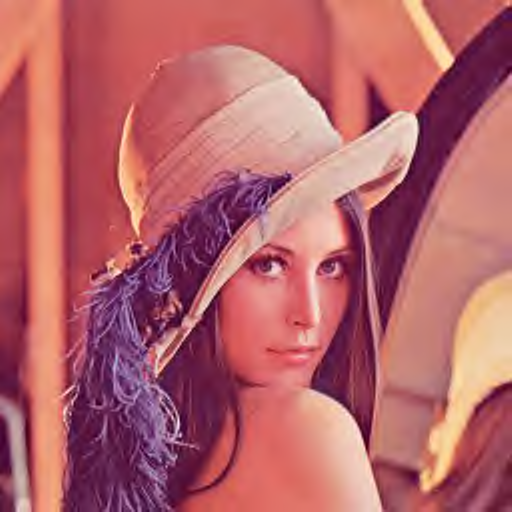• Spatial scalability: More decoded data, more resolution.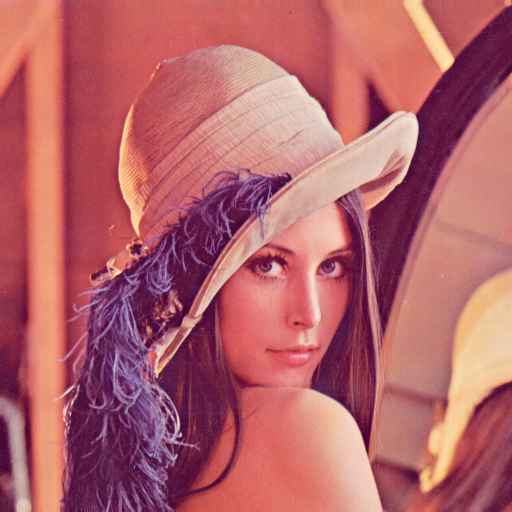• ROI (Regions Of Interest) scalability:### 3 The JPEG2000 algorithm1. Color component DC level oﬀset (optional).
2. Intercomponent decorrelation (optional).
3. Spatial decorrelation (2D-DWT).
4. Quantization (only in the lossy path).
5. ROI deﬁnition.
6. Entropy coding (tier-1 coding).
7. Bit-stream organization (tier-2 coding).

### 4 DC level oﬀset (step 1/6)

• Depends on the selected path. For each component:
1. Irreversible: normalize the samples $s\left[\text{}n\text{}\right]$ in order to satisfy that  $-\frac{1}{2}\le s\left[\text{}n\text{}\right]\le \frac{1}{2},$ (1)

where $s\left[\text{}n\text{}\right]=s\left[x,y\right]$ is a point.

2. Reversible: substract an oﬀset to $s\left[\text{}n\text{}\right]$ if they does not verify than  $-{2}^{B-1}\le s\left[\text{}n\text{}\right]<{2}^{B-1},$ (2)

where $B$ is the number of bits/component.

### 5 Component decorrelation (step 2/6)

• Only to color (RGB) images.
1. Irreversible path:  $\begin{array}{c}\text{Y}=0.299\text{R}+0.587\text{G}+0.144\text{B}\hfill \\ \phantom{\rule{0.33em}{0ex}}\hfill \\ \text{Cb}=\frac{0.5}{1-0.144}\left(\text{B}-\text{Y}\right)\hfill \\ \phantom{\rule{0.33em}{0ex}}\hfill \\ \text{Cr}=\frac{0.5}{1-0.299}\left(\text{R}-\text{Y}\right)\hfill \end{array}$ (3)
2. Reversible path:  $\begin{array}{c}\text{Y´}=⌊\frac{\text{R}+2\text{G}+\text{B}}{4}⌋\hfill \\ \phantom{\rule{0.33em}{0ex}}\hfill \\ \text{Db}=\text{B}-\text{G}\hfill \\ \phantom{\rule{0.33em}{0ex}}\hfill \\ \text{Dr}=\text{R}-\text{G}\hfill \end{array}$ (4)

### 6 The 2D DWT (step 3/6)

• In JPEG 2000, the 2D DWT is applied independently to each component.
1. Irreversible path:  $\begin{array}{c}\hfill \begin{array}{cc}\multicolumn{2}{c}{L\left(z\right)=}\\ \hfill & 0.602949018236\hfill \\ +\hfill & 0.266764118443\left({z}^{1}+{z}^{-1}\right)\hfill \\ -\hfill & 0.078223266529\left({z}^{2}+{z}^{-2}\right)\hfill \\ -\hfill & 0.016864118443\left({z}^{3}+{z}^{-3}\right)\hfill \\ +\hfill & 0.026748757411\left({z}^{4}+{z}^{-4}\right)\hfill \end{array}\hfill \\ \hfill \hfill \\ \hfill \begin{array}{cc}\multicolumn{2}{c}{H\left(z\right)=}\\ \hfill & 0.557543526229\hfill \\ +\hfill & 0.295635881557\left({z}^{1}+{z}^{-1}\right)\hfill \\ -\hfill & 0.028771763114\left({z}^{2}+{z}^{-2}\right)\hfill \\ -\hfill & 0.045635881557\left({z}^{3}+{z}^{-3}\right)\hfill \end{array}\hfill \end{array}$ (CDF 9/7)

2. Reversible path:  $\begin{array}{c}H\left(z\right)=-\frac{1}{8}\left({z}^{2}+{z}^{-2}\right)+\frac{1}{4}\left({z}^{1}+{z}^{-1}\right)+\frac{3}{4}\hfill \\ \phantom{\rule{0.33em}{0ex}}\hfill \\ L\left(z\right)=-\frac{1}{2}\left({z}^{1}+{z}^{-1}\right)+1\hfill \end{array}$ (Spline 5/3)

### 7 Quantization (step 4/6)

#### Irreversible path

 ${q}_{b}=\text{sign}\left({y}_{b}\right)⌊\frac{|{y}_{b}|}{{\Delta }_{b}}⌋$ (J2KQuant)

where ${q}_{b}$ is the quantized coeﬃcient, ${y}_{b}\in \left[-0.5,0.5\right]$ is a wavelet coeﬃcient in the subband $b$ and ${\Delta }_{b}$ is the quantizer step size for the subband $b$, whose value depends on ${y}_{b}$ as it is shown in the next ﬁgure (deathzone scalar quantizer):#### Reversible path

There is no quantization:

 ${q}_{b}={y}_{b}$ (J2KRanging)

### 8 ROI deﬁnition (step 5/6)

• Obtained by prioritizing (multiplying by a number greater than one) those ${q}_{b}$ that deﬁne the ROI.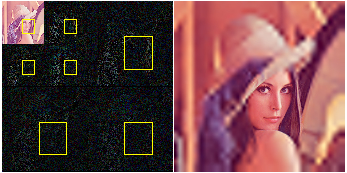### 9 Entropy encoding (step 6/6)

1. EBCOT (Embedded Block Coding with Optimal Truncation).
2. PCRD-opt (Post Compression Rate Distortion optimization).

#### EBCOT 

• The coeﬃcients are grouped into code-blocks (that have a typical size of $32×32$ or $64×64$) and encoded bit-plane by bit-plane, using a context-based adaptive binary arithmetic encoder (called MQ-coder).• Each bit-plane of each code-block is encoded in 3 passes:
1. Signiﬁcance propagation pass: indicates if the coeﬃcients that are expected to be signiﬁcant (in absolute value larger than ${2}^{p}$, where $p$ is the index of the processed bit-plane), are signiﬁcant in fact. When a coeﬃcient becomes signiﬁcant, its sign is also encoded.
2. Magnitude reﬁnement pass: indicates the correspondent bit value for the processed bit-plane for those coeﬃcients that, already, are signiﬁcant.
3. Cleanup pass: the signiﬁcance propagation only determines a subset of the total coeﬃcients that can become signiﬁcant. This pass solves this problem.
• The code-stream produced after each individual pass is an optimal code-stream from the R/D point of view. In other words, if the code-stream is truncated at any of these points, we are the closest to the R/D curve as it is possible.• Notice that there are a total of  $3P-2$ (5)

optimal truncation points in the code-stream of a code-block, where $P$ is the number of bit-planes in the DWT domain.

### PCRD-opt

• In order to provide quality scalability, the code-stream of the code-blocks should be shuﬄed attending to the contribution of each coding pass to the increment of quality of the reconstruction of the whole image.
• A JPEG 2000 encoder typically inputs a set of $Q$ bit-rates or a number of $Q$ quality layers.
• The PCRD-opt determines which segments of each code-block-stream are going to be part of a quality layer. Example:### The precinct partition

• Unfortunately, there is no a single code-stream ordering that generates both scalabilities: spatial and quality.
• Therefore, when the data-ordering in the code-stream does not match with the target scalability, the only solution is to access to the code-stream using a non-sequential ordering. For this reason, some extra data (overhead) should be included in the code stream (remember that the contribution (in bits of code) to each code-block to the total quality can be diﬀerent).
• Finally, if $Q$ is high, the amount of overhead could be counterproductive.
• To mitigate this drawback, the code-blocks (and their code-streams) are grouped into the so called precincts.
• So, each “quality layer” of each precinct is stored in a packet and there is a index (or a length) for each packet in a JPEG 2000 code-stream.### Reduction of the distortion of a coding pass

• The contribution to the quality (distortion decrease) of a coding pass to the total distortion of the reconstruction is determined by:
1. The weight of the bits of the coeﬃcients that are encoded in the coding pass.
2. The energy gain factor of the subband where the code-block is located.

### Progressions of JPEG2000

• In fact, a JPEG 2000 codec produces a packet for each precinct, component, resolution level and quality layer.
• Depending on the ﬁnal packet ordering, we have one of the following progressions:

### LRCP or quality progression

• Packets are ordered ﬁrst by quality, then by resolution level, then by component and ﬁnally, by precinct. Example:### RLCP or spatial progression

• Packets are ordered ﬁrst by resolution level, then by quality layer, then by component and ﬁnally, by precinct. Example:### PCRL or sequential progression

• Packets are ordered ﬁrst by precinct, then by component, after that by spatial resolution and ﬁnally, by quality layer. Example:### Rudimentary ROI deﬁnition at decoding time

• The random access to the packet-stream give the possibility of the deﬁnition of a ROI by the decoder.
• The accuracy of the shape of the ROI depends on the precinct size(s). The smaller the precincts, the better the precision.
• This feature is typically exploited in client/server architectures through the JPIP (JPeg 2000 Interactive Protocol) .

### “Lena” at $0.1$ bpp

 JPEG ($21.29$ dB) JPEG2000 ($27.03$ dB)### “Lena” at $0.2$ bpp

 JPEG ($26.64$ dB) JPEG2000 ($29.22$ dB)### “Lena” at $0.3$ bpp

 JPEG ($28.97$ dB) JPEG2000 ($30.71$ dB)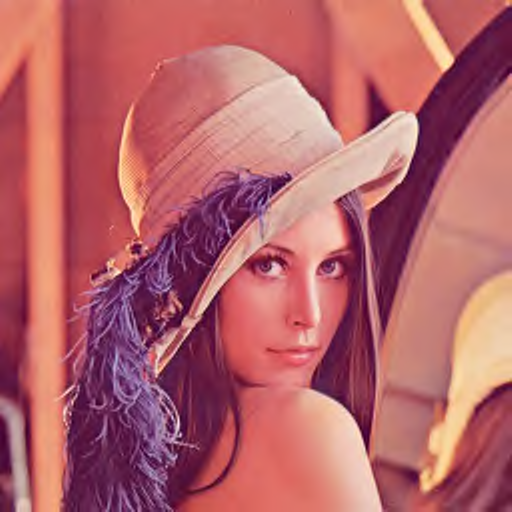### “Lena” at $0.4$ bpp

 JPEG ($30.09$ dB) JPEG2000 ($31.58$ dB)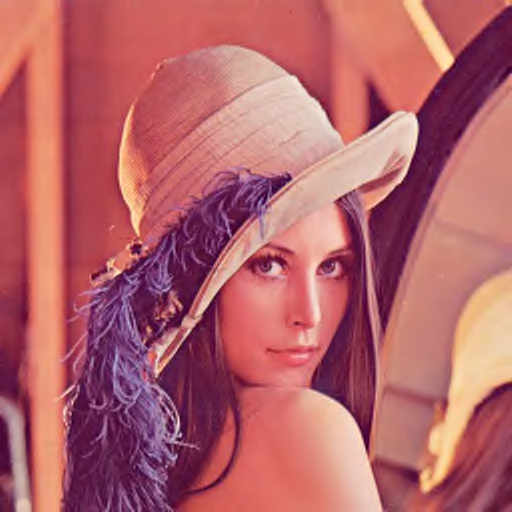### “Lena” at $0.5$ bpp

 JPEG ($30.91$ dB) JPEG2000 ($32.24$ dB)### “Cat” at $0.1$ bpp

 JPEG ($17.79$ dB) JPEG2000 ($23.33$ dB)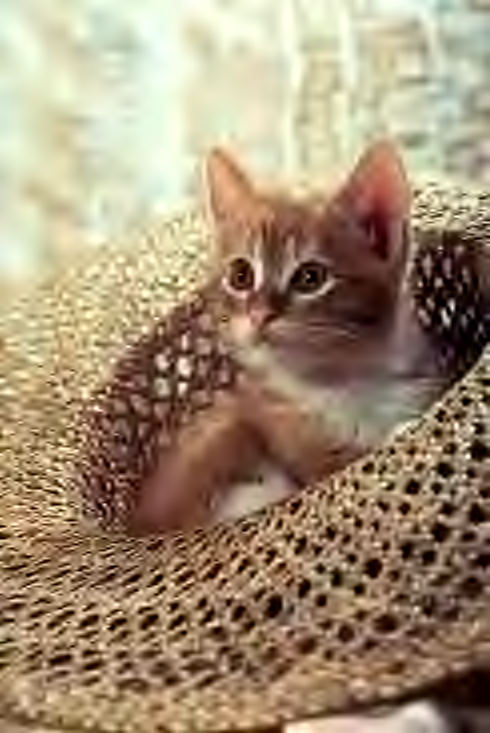### “Cat” at $0.2$ bpp

 JPEG ($23.59$ dB) JPEG2000 ($25.97$ dB)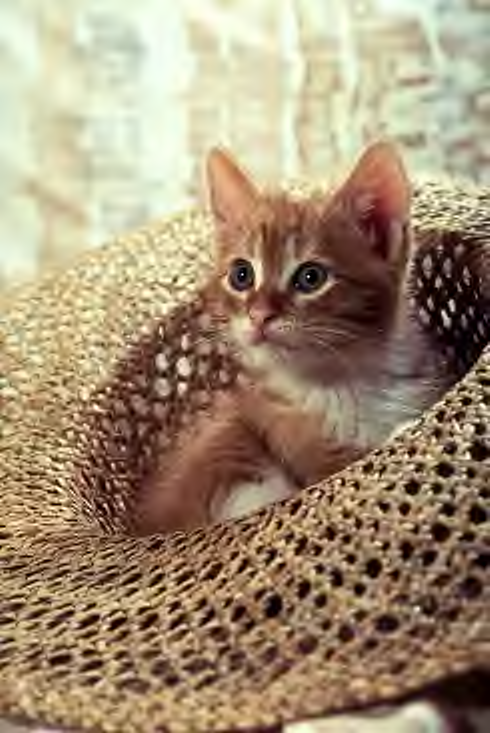### “Cat” at $0.3$ bpp

 JPEG ($25.63$ dB) JPEG2000 ($27.60$ dB)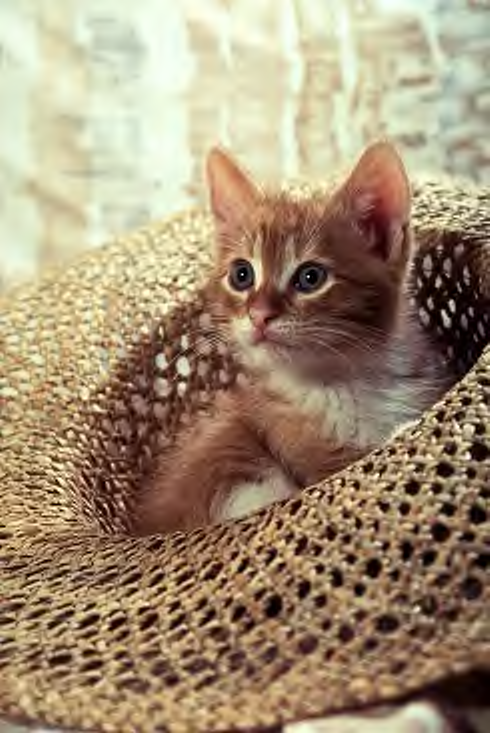### “Cat” at $0.4$ bpp

 JPEG ($27.10$ dB) JPEG2000 ($28.97$ dB)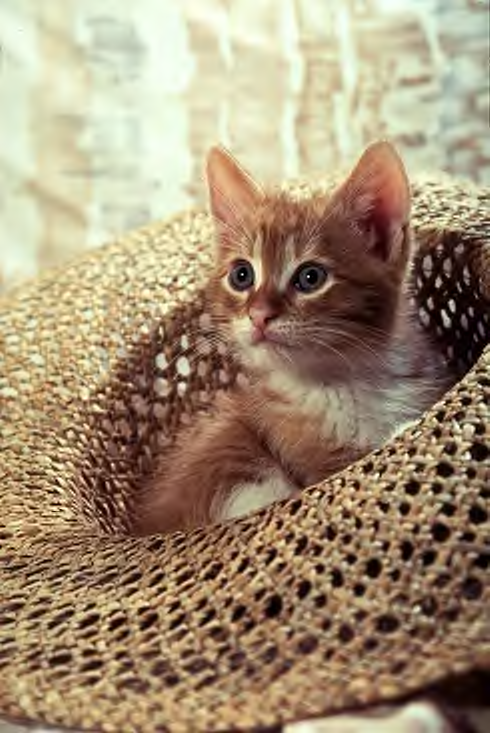### “Cat” at $0.5$ bpp

 JPEG ($28.23$ dB) JPEG2000 ($30.08$ dB)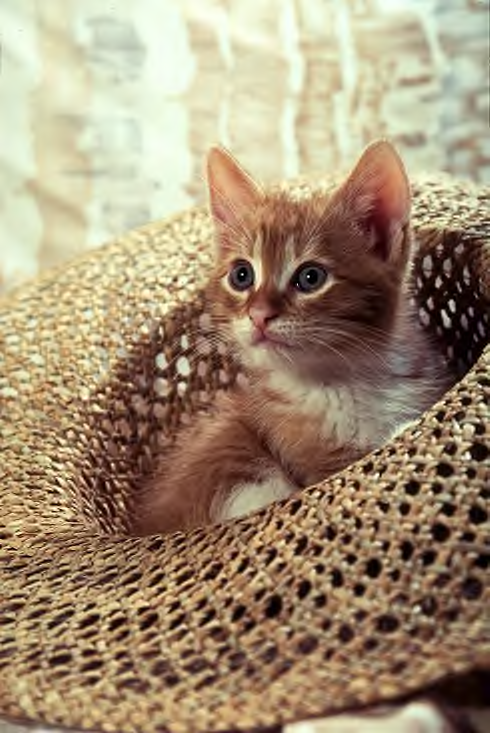### 10 Motion JPEG 2000

• As in JPEG, JPEG 2000 has an extension  to compress sequences of images.
• Each image is encoded independently.
• But at diﬀerence of JPEG, the code-streams can be variable bit-rate (compression ratio selected by slope(s)) or constant bit-rate (compression ratio selected by bit-rate(s)).
• Finally, scalability can be used to recover a reduced quality, lower ROI/resolution or gray-scale version of the original image.